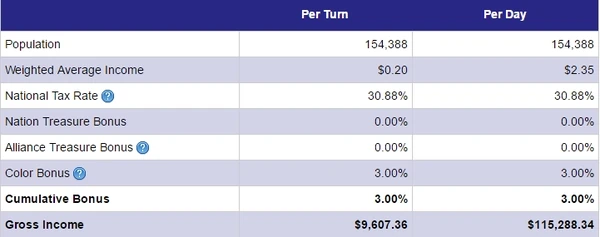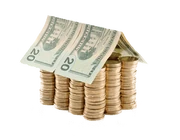## FANDOM

1,706 Pages

Formulas relating to a nation.

## Average IncomeEdit

Minimum Wage

```Minimum Wage = 725/(Tax Rate * 1000)
```

Average Income

```Average Income = ((Commerce/50) * Minimum Wage) + Minimum Wage
```

Merging both together will give

```Average Income = ((Commerce/50) * (725/(Tax Rate * 1000))) + (725/(Tax Rate * 1000))
```

(Formulas give values for daily average income)

Daily City Income

```City Income = (((Commerce/50) * 0.725) + 0.725) * Population
```

(Formula ignores tax rates)

## Gross Income FormulaEdit

The Gross Income can be calculated by the following formula:

``` Gross Income = Weighted Average Income * Population * Tax Rate
```

Where

Additional factors that further affect the Gross Income are:

• If your Domestic Policy is Open Markets: Gross Income = Gross Income * 1.01
• If your nation is out of food: Gross Income = Gross Income * 0.67
• If you or your alliance have Treasure/s then there will be an additional bonus

## Nation Score FormulasEdit

The formula is:

``` Nation Score = (City Count - 1) *100 + (Nation Infra Total/40) + (Project Count *20) + ((Soldiers * 0.0004) + (Tanks * 0.009) + (Aircraft * 0.2) + (Ships * 0.75) + (Missiles * 5) + (Nuclear Weapons * 15)) + 10
```

Or Simplified

```Nation Score = City Score + Infrastructure Score + Project Score + Military Score + Base Score
```

### City scoreEdit

```City Score = (Number Of Cities - 1) * 100
```

### Infrastructure ScoreEdit

```Infrastructure Score = Nation Infrastructure Total/40
```

### Project ScoreEdit

```Project Score = Number Of Projects * 20
```

### Military ScoreEdit

```Military Score = (Soldiers * 0.0004) + (Tanks * 0.009) + (Aircraft * 0.2) + (Ships * 0.75) + (Missiles * 5) + (Nuclear Weapons * 15)
```

Score from Missiles and Nuclear Weapons are capped at 50 each (the 51st Missile or Nuclear Weapon will not add to your nation score.)

### Base ScoreEdit

```Base Score = 10
```

## War RangeEdit

A nations war range is used to determine the nations that you can or cannot attack, this is determined by your own nation score.

### Min Score FormulaEdit

```Score = Your Nation Score * 0.75
```

### Max Score FormulaEdit

```Score = Your Nation Score * 1.75
```

### Inverse Formula'sEdit

These formulas are used to find the nation score from the lowest or highest score.

#### Has MinEdit

```Nation Score = (Min Score / 25) * 100
```

#### Has MaxEdit

```Nation Score = Max Score / 1.75
```

## Temp -- talk to zevferEdit

for storage -- will be moved/used somewhere else laterThe Gross Income is how much money your nation makes before any expenses are paid.

Community content is available under CC-BY-SA unless otherwise noted.